# Archive | February, 2016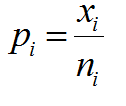## P Chart with Minitab

What is a P Chart? The P chart plots the percentage of defectives in one subgroup as a data point. It considers the situation when the subgroup size of inspected units is not constant. The underlying distribution of the P-chart is binomial distribution. P Chart Equations Data Point:         Center Line:   [...]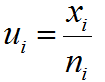## U Chart with Minitab

What is a U Chart? The U chart is a type of control chart used to monitor discrete (count) data where the sample size is greater than one, typically the average number of defects per unit. Defect vs. Defective Remember the difference between defect and defective? A defect of a unit is the unit’s characteristic [...]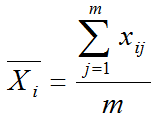## Xbar R Chart with Minitab

Xbar R Chart The Xbar R chart is a control chart for continuous data with a constant subgroup size between two and ten. The Xbar chart plots the average of a subgroup as a data point. The R chart plots the difference between the highest and lowest values within a subgroup as a data point. [...]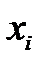## IR Chart with Minitab

What is an IR Chart? The IR chart (also called individual-moving range chart or I-MR chart) is a popular control chart for continuous data with subgroup size equal to one. The I chart plots an individual observation as a data point. The MR chart plots the absolute value of the difference between two consecutive observations [...]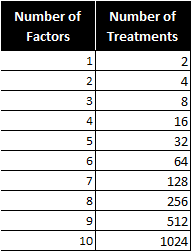## Fractional Factorial Designs with Minitab

What Are Fractional Factorial Experiments? In simple terms, a fractional factorial experiment is a subset of a full factorial experiment. Fractional factorials use fewer treatment combinations and runs. Fractional factorials are less able to determine effects because of fewer degrees of freedom available to evaluate higher order interactions. Fractional factorials can be used to screen [...]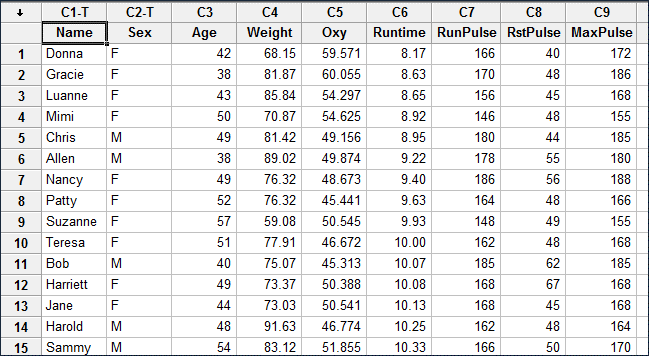## Stepwise Regression in Minitab

What is Stepwise Regression? Stepwise regression is a statistical method to automatically select regression models with the best sets of predictive variables from a large set of potential variables. There are different statistical methods used in stepwise regression to evaluate the potential variables in the model: Three Approaches to Stepwise Regression How to Use Minitab […]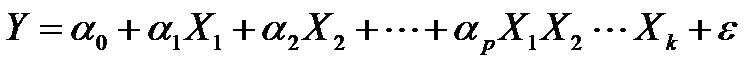## Full Factorial with Minitab

What is a Full Factorial DOE? In a full factorial experiment, all of the possible combinations of factors and levels are created and tested. For example, for two-level design (i.e.each factor has two levels) with k factors, there are 2k possible scenarios or treatments. Two factors, each with two levels, we have 22 = 4 treatments […]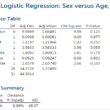## Logistic Regression with Minitab

What is Logistic Regression? Logistic regression is a statistical method to predict the probability of an event occurring by fitting the data to a logistic curve using logistic function. The regression analysis used for predicting the outcome of a categorical dependent variable, based on one or more predictor variables. The logistic function used to model […]